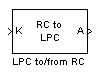# LPC to/from RC

Convert linear prediction coefficients to reflection coefficients or reflection coefficients to linear prediction coefficients

## Library

Estimation / Linear Prediction

`dsplp`

•## Description

The LPC to/from RC block either converts linear prediction coefficients (LPCs) to reflection coefficients (RCs) or reflection coefficients to linear prediction coefficients. Set the Type of conversion parameter to `LPC to RC` or `RC to LPC` to select the domain into which you want to convert your coefficients. The A port corresponds to LPC coefficients, and the K port corresponds to the RC coefficients. For more information, see Algorithm.

The block input can be an N-by-M matrix or an unoriented vector. Each column of the matrix is treated as a channel. When the input is an unoriented vector, the input is treated as one channel.

Consider a signal x (n) as the input to an FIR analysis filter represented by LPC coefficients. The output of the analysis filter, e (n), is known as the prediction error signal. The power of this error signal is denoted by P. When the zero lag autocorrelation coefficient of x (n) is one, the autocorrelation sequence and prediction error power are said to be normalized.

Select the Output normalized prediction error power check box to enable port P. The normalized prediction error power output at `P` is a vector with one element per input channel. Each element varies between zero and one.

Select the Output LPC filter stability check box to output the stability of the filter represented by the LPCs or RCs. The synthesis filter represented by the LPCs is stable when the absolute value of each of the roots of the LPC polynomial is less than one. The lattice filter represented by the RCs is stable when the absolute value of each reflection coefficient is less than 1. When the filter is stable, the block outputs a Boolean value of `1` for each input channel at the S port. When the filter is unstable, the block outputs a Boolean value of `0` for each input channel at the S port.

If first input value is not 1 parameter specifies the behavior of the block when the first coefficient of the LPC coefficient vector in any channel is not 1. The following options are available:

• `Replace it with 1` — Changes the first value of the coefficient channel to 1. The other coefficient values are unchanged.

• `Normalize` — Divides the entire channel of coefficients by the first coefficient so that the first coefficient of the LPC coefficient vector is 1.

• `Normalize and Warn` — Divides the entire channel of coefficients by the first coefficient so that the first coefficient of the LPC coefficient vector is 1. The block displays a warning message telling you that your vector of coefficients has been normalized.

• `Error` — Displays an error telling you that the first coefficient of the LPC coefficient channel is not 1.

## Algorithm

### LPC to RC

When in this mode, this block uses backward Levinson recursion to convert linear prediction coefficients (LPCs) to reflection coefficients (RCs). For a given Nth order LPC vector $LP{C}_{N}=\left[\begin{array}{ccccc}1& {a}_{N1}& {a}_{N2}& \dots & {a}_{NN}\end{array}\right]$, the block calculates the Nth reflection coefficient value using the formula ${\gamma }_{N}=-{a}_{NN}$. The block then finds the lower order LPC vectors , , using the following recursion.

for p = N, N – 1, ..., 2,

end

Finally, ${\gamma }_{1}=-{a}_{11}$. The reflection coefficient vector is $\left[\begin{array}{cccc}{\gamma }_{1},& {\gamma }_{2},& \dots ,& {\gamma }_{N}\end{array}\right]$.

### RC to LPC

When in this mode, this block uses Levinson recursion to convert reflection coefficients (RCs) to linear prediction coefficients (LPCs). In this case, the input to the block is $RC=\left[\begin{array}{cccc}{\gamma }_{1}& {\gamma }_{2}& ...& {\gamma }_{N}\end{array}\right]$. The zeroth order LPC vector term is 1. Starting with this term, the block uses recursion to calculate the higher order LPC vectors, $LP{C}_{2},LP{C}_{3},...LP{C}_{N}$, until it has calculated the entire LPC matrix.

`$LP{C}_{matrix}=\left[\begin{array}{c}LP{C}_{0}\\ LP{C}_{1}\\ LP{C}_{2}\\ \cdots \\ LP{C}_{N}\end{array}\right]=\left[\begin{array}{cccccc}1& 0& 0& 0& \cdots & 0\\ 1& {a}_{11}& 0& 0& \cdots & 0\\ 1& {a}_{21}& {a}_{22}& 0& \cdots & 0\\ 1& {a}_{31}& {a}_{32}& {a}_{33}& \cdots & 0\\ \cdots & \cdots & \cdots & \cdots & \cdots & \cdots \\ 1& {a}_{N1}& {a}_{N2}& {a}_{N3}& \cdots & {a}_{NN}\end{array}\right]$`

This LPC matrix consists of LPC vectors of order 0 through N found by using the Levinson recursion. The following are the formulas for the recursion steps, for p = 0, 1, ...,N – 1.

## Parameters

Type of conversion

Select `LPC to RC` or ```RC to LPC``` to select the domain into which you want to convert your coefficients.

Output normalized prediction error power

Select this check box to output the normalized prediction error power at port P.

Output LPC filter stability

Select this check box to output the stability of the filter. When the filter represented by the LPCs or RCs is stable, the block outputs a Boolean value of `1` for each input channel at the S port. When the filter represented by the LPCs or RCs is unstable, the block outputs a Boolean value of `0` for each input channel at the S port.

If first input value is not 1

Select what you would like the block to do when the first coefficient of the LPC coefficient vector is not 1. You can choose ```Replace it with 1```, `Normalize`, `Normalize and Warn`, and `Error`.

## References

Makhoul, J Linear Prediction: A tutorial review. Proc. IEEE. 63, 63, 56 (1975).

Markel, J.D. and A. H. Gray, Jr., Linear Prediction of Speech. New York, Springer-Verlag, 1976.

## Supported Data Types

• Double-precision floating-point

• Single-precision floating-point